# Overview

This vignette demonstrates the Dynamic Energy Budget (DEB) model functions of the NicheMapR package (Kearney and Porter, 2019). The package includes stand-alone R functions for simulating DEB models as well as the integration of DEB models with the NicheMapR ectotherm model (see Introduction to the NicheMapR ectotherm model).

The document first provides a short overview of DEB theory in the context of mechanistic niche modelling, and describes the modelling scheme overall and the parameters required. It then shows how to bring existing DEB parameter estimates into R (there are now estimates for ~1,800 species) and how to run simulations from them using the DEB R functions within the package. Finally it demonstrates how to use the DEB model within the NicheMapR ectotherm function to predict the full life-cycle heat, water, energy and nutritional budget as a function of microclimate.

DEB theory is a thermodynamically formalised framework for capturing the ‘macro-metabolic’ processes of feeding, assimilation, storage, mobilisation, maintenance, development, growth, reproduction and senescence of an organism across the whole life cycle. It was developed by Kooijman over ~30 years (Kooijman 1986a, 1986b, 1993, 2000, 2010). There is a family of models under DEB theory which capture qualitatively different metabolic schemes, including ‘von Bertalanffy growth’ and more complex patterns such as those of holometabolus insects (Llandres et al. 2015).

Earlier versions of the ‘Niche Mapper’ biophysical modeling framework (e.g. Porter et al. 1973; Kearney and Porter 2004) incorporated ‘static energy budgets’, i.e. considering only one particular life stage a snapshot in time, using descriptive empirical functions of metabolic processes such as allometric functions for metabolic rate. DEB theory provides a more mechanistic basis for doing this dynamically through the ontogeny, thereby capturing the temporal dimension of the niche.

There are natural feedbacks between the biophysical and DEB models including the interaction between size, heat and water exchange, the interaction between feeding and activity, and the interaction between metabolism and the water budget. It thereby provides a holistic picture of the heat, water, energy and nutritional budget and its life history consequences in a given sequence of environments.

# Example DEB simulation

First let’s take a look at what comes out of a DEB model. Figure 1 shows the prediction of length through time under the ‘standard’ DEB model for the Australian lizard, the Eastern Water Skink, Eulamprus quoyii.

In this simulation the lizard is growing at constant temperature (25 °C) and ad libitum constant food. Under these conditions, the DEB standard model equates to the von Bertalanffy growth equation from the point of birth. Note that the reasoning that leads to von Bertalanffy growth being a special case of DEB theory is not the same as that used by Pütter (1920) to derive the original ‘von Bertalanffy’ growth equation.

Before birth, growth is faster than what the von Bertalanffy equation would predict because of the DEB assumptions of embryonic growth. These assumptions, simply put, are that an embryo is the same as a hatchling metabolically speaking except that it does not feed, and that the concentration of the nutritive substance (‘reserve’) has started off extremely high, and has not reached its steady state concentration (technically a steady state density). The initial concentration is extremely high because the lizard starts off as a tiny structure (fertilised cell) with a large amount of yolk, i.e. reserve. Once the animal is born, i.e. starts feeding, reserve reaches a steady state, and the von Bertalanffy-like growth dynamics ensue.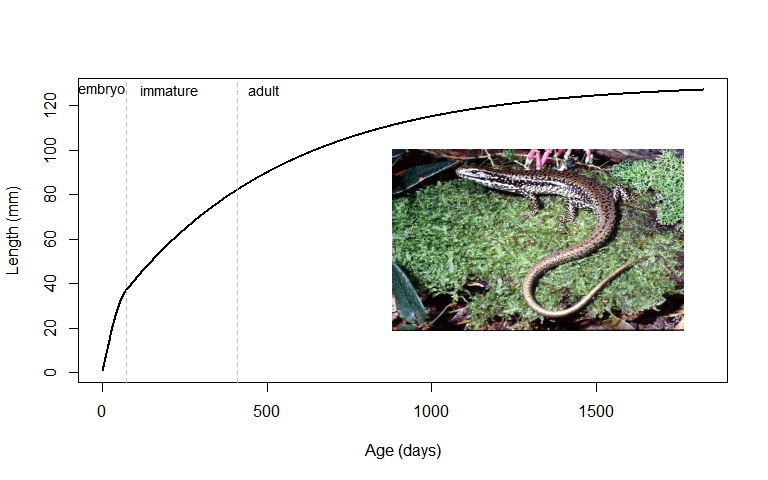DEB model prediction of length through time of the lizard Eulamprus quoyii growing under constant, ad libitum food at 25 °C. Photo by the author.

The next plot shows the growth in mass. In DEB theory, biomass is partitioned into three types, reserve, structure and reproduction buffer. The first two were mentioned already in the discussion of length, above. Reserve should be though of as stored metabolites in the form of polymers (proteins, lipids, carbohydrates) that are made by the process of assimilation, and mobilised to run the metabolism. The structure is what is built from the reserve and the amount of structure is proportional to the cube of the length of the organism. A given cell in the organism should be conceived as being part reserve and part structure. An egg is pure reserve. The process of reproduction is the build up of material in the body that is the same composition as reserve and packaged up into eggs to be released into the environment.

In Figure 2, the partitioning of the total biomass is indicated by the different coloured lines, starting with the structure in green, then adding on top of that the reserve, and then on top of that the reproduction buffer. You can also see in brown the food in the gut which of course also contributes to the wet weight of an animal.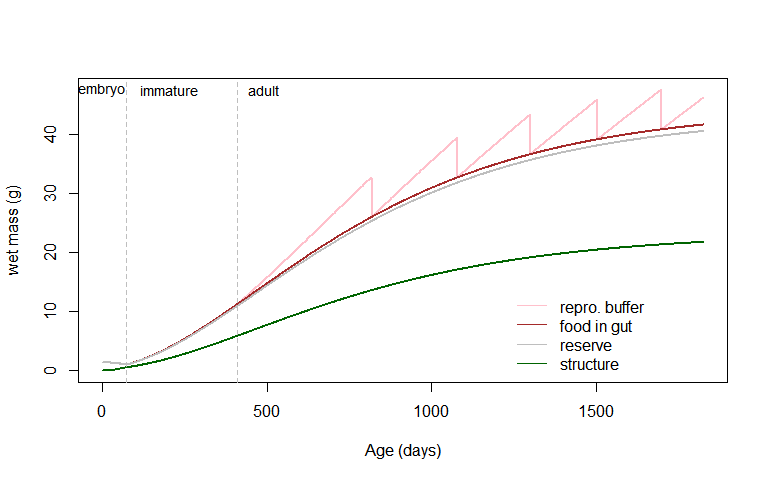DEB model prediction of wet weight through time of the lizard Eulamprus quoyii growing under constant ad libitum food at 25 °C, partitioning total biomass mass into structure, reserve, reproduction buffer and gut contents.

Note in Figure 2 how the embryo starts off as near zero structure and is mostly reserve, and that the overall mass declines through development but the structure goes up. Also note that the point of maturity is where the reproduction buffer starts to grow, but the time of first clutch (the sudden drops in mass reflecting the eggs being released to the environment) is some time later once enough reproduction has been accumulated.

# The DEB scheme

Only a very cursory discussion of the rationale behind the DEB theory can be provided here, and it is recommended that the reader consults the core DEB references already mentioned (Kooijman 1986a, Kooijman 1986b, Kooijman 1993, Kooijman 2000, Kooijman 2010 – this is a very good order to read them in too).

Figure 3 summarises the DEB scheme, showing the network of macro-metabolic processes considered (but not including aging). The state variables are represented by the boxes. In a non-embryo, feeding occurs, which is the transformation of food into reserve through the process of assimilation. The reserve is then mobilised to fuel growth, maintenance, maturation, maturity maintenance and reproduction.

The mobilisation rate depends on the concentration (density) of the reserve as well as the inherent energy conductance of the organism. You should think of the reserve as blobs of polymers in a matrix of structure that need to be accessed from the periphery, and it is this surface area/volume interaction between reserve and structure that determines the dynamics of mobilisation. Also, if you imagine enzymes eating away at the surface of the blobs of reserve, the density of those enzymes (or their speed of action) would represent the energy conductance. The reserve dams up according to both the rate of assimilation, and also the rate of mobilisation. The energy conductance in part determines the pace of life.

Mobilised reserve (energy as well as mass for building blocks) is partitioned according to the parameter kappa $$\kappa$$, so that a certain fraction goes to the processes of somatic growth and somatic maintenance. Growth is the synthesis of structure from the reserve, and requires energy to do the work of building as well as the energy and matter (and water) that goes into the newly made structural biomass. This structure requires energy for maintenance in direct proportion to how much structural biomass has been made, and maintenance takes priority over growth. The mobilisation rate of the reserve is proportional to the surface area of the structure, and so increases more slowly than the requirements for maintenance. Thus growth slows down through time as the organism approaches an asymptotic maximum size that reflects the point where everything going down the branch to growth and maintenance is consumed by maintenance. This is how the von Bertalanffy growth curve emerges from DEB theory.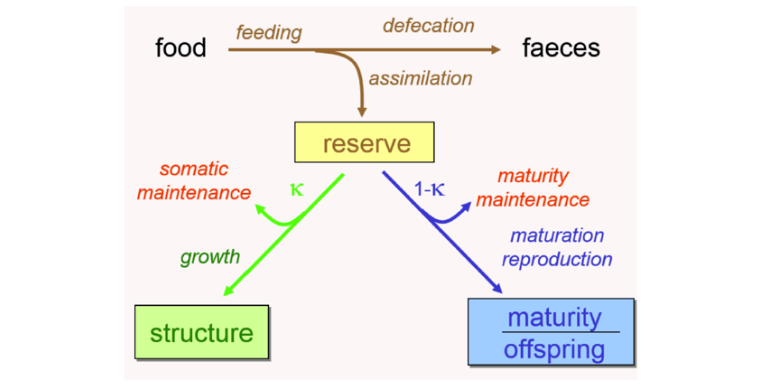Stucture of the standard model of Dynamic Energy Budget theory, from Marques et al. (2018). Boxes represent state variables, arrows represent processes.

The other branch of mobilised reserve, $$1-\kappa$$, initially goes to the process of ‘maturation’. This process is the use of energy to make the organism more complex as it develops. It also must invest energy in ‘maturity maintenance’, i.e. to stay at the current level of maturity. This is analogous to somatic maintenance, and the metaphor to keep in mind is that of needing to practice a new language that you have learned to keep you from forgetting it. At some threshold of energy investment into maturation, the organism has reached ‘puberty’. From this point onward, any surplus energy going down the $$1-\kappa$$ branch, once the cost of maturity maintenance has been paid, goes into the reproduction buffer.

As mentioned above, the embryo follows the same scheme but does not feed and starts off at a non-steady-state reserve level of extremely high density.

A very powerful aspect of the DEB theory is that it allows the mass budget to be computed on an elemental basis. A core assumption of DEB theory, ‘strong homeostasis’, is that the biomass pools that the organism is abstracted into, i.e. structure, reserve, reproduction buffer, have a constant chemical composition. This means they can be thought of as ‘macro-molecules’, and one can write out equations for the transformation of these molecules from one form to another. Without this abstraction of biomass into stoichiometrically fixed pools, one cannot obtain an elemental balance.

In the simplest case, there are four ‘organic’ molecules, the food $$X$$, the structure $$V$$, the reserve $$E$$ and the faeces $$P$$, and four ‘mineral’ molecules: oxygen, carbon dioxide, water and a nitrogenous waste product such as ammonia or uric acid. Because most of the biomass is made up of the four elements, carbon, hydrogen, oxygen and nitrogen, for most purposes only these need to be considered. And the ‘organic’ molecules can be specified in terms of the proportions of hydrogen, oxygen and nitrogen relative to the amount of carbon, i.e. in ‘c-moles’.

Figure 4 shows the mass budget scheme used in the DEB theory. The symbol $$\dot{J}$$ means mass, the symbol $$\dot{p}$$ represents energy flow (power), and the dot above means a flux (per time). The elemental composition of the different molecules is represented by $$n_{1,2}$$ where 1 represents the element (C, H, O or N) and 2 represents the ‘molecule’ (e.g. $$V$$ for structure or $$C$$ for $$CO_2$$). The fluxes (moles per time) of food, structure, reserve and faeces.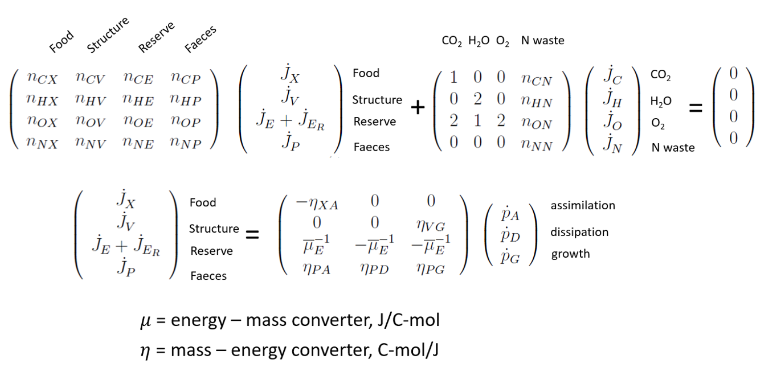Stoichiometric equations of the standard model of Dynamic Energy Budget theory, taken from Kooijman (2010).

The first equation in Figure 4 is simply a statement of the conservation of mass; the flows of all the elements in the different metabolic processes, when added together, must sum to zero. The second equation states the relationship between the four organic mass fluxes and the three energy fluxes of assimilation, growth and ‘dissipation’ (maturation and its maintenance, somatic maintenance, any heating or osmoregulation costs, and reproduction overhead costs), given the conversion coefficent matrix for energy/mass.

The key point is that knowing the energy fluxes, computed from the DEB model as it evolves through time, and the stoichiomeric composition of food, structure, reserve and faeces, one can compute the oxygen, carbon dioxide, metabolic water and nitrogenous waste fluxes. Although we don’t usually have explicit data on these elemental compositions, the default values [based on general estimates of organismal stoichiometry, e.g. Roels (1983)] give good predictions in practice. Thus, by producing a DEB model we can get realistic dynamics of development, growth and reproduction under fluctuating temperature and food scenarios, and we also get estimates of the fluxes of food, faeces, metabolic water, respiratory gases and nitrogenous waste production ‘for free’, as shown in Figure 5 for Eulamprus quoyii. This is one of the many pay-offs for abstracting biomass into pools of reserve and structure.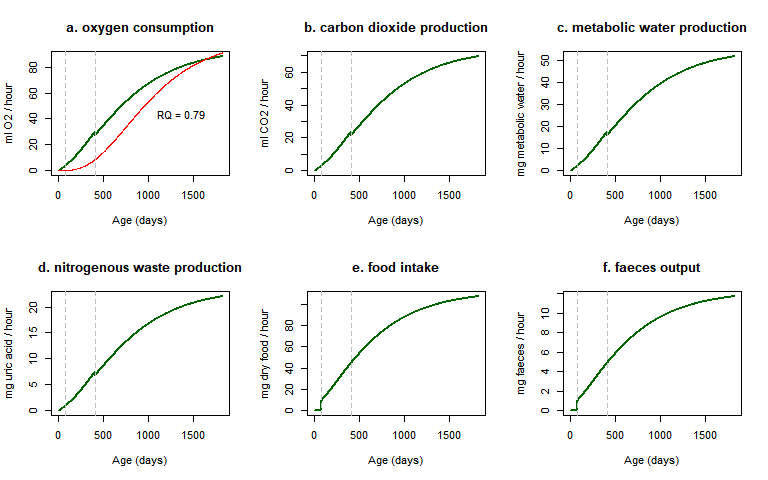DEB predictions of some mass fluxes for the lizard Eulamprus quoyii growing under constant ad libitum food at 25 °C. In panel a, the red line is the prediction from a general allometric relation for lizards (Andrews and Pough 1985). ‘RQ’ is the respiratory quotient, the ratio of $$CO_2$$ to $$O_2$$ production.

# The DEB parameters required for a full energy and mass budget

To run a DEB simulation you of course need some DEB parameters. The number of parameters required depends on the number of processes being made explicit in the DEB model. Minimally, one needs the following seven ‘core’ parameters to predict the dynamics of just the state variables structure, reserve and maturity/reproduction, at one temperature and one food level, across the whole life cycle (Table 1 – this table excludes the parameters $$k_J$$, the maturity maintenance rate coefficient, and $$\kappa_R$$, the conversion efficiency of reproduction buffer to eggs, which are usually left as default values because they are difficult to estimate and the plausible range of values typically has low influence on the predictions).

Table 1. Minimal required core parameters of the standard DEB model.
Parameter Parameter Symbol Unit
max surface-specific assimilation rate $$\{\dot{p}_{Am}\}$$ $$J\:cm^{-2}\:d^{-1}$$
energy conductance $$\dot{v}$$ $$d^{-1}$$
allocation fraction to soma $$\kappa$$ -
volume-specific somatic maintenance cost $$[\dot{p}_{M}]$$ $$J\:cm^{-3}\:d^{-1}$$
volume-specific cost for structure $$[E_g]$$ $$J\:cm^{-3}$$
maturity at birth $$E_{H}^{b}$$ $$J$$
maturity at puberty $$E_{H}^{p}$$ $$J$$

These parameters relate to the abstract state variables, i.e. they don’t represent measurable ‘real-world’ quantities. However, because they have a one-to-many relationship with certain life history traits, it is possible to obtain good estimates through inversely fitting them to such observations. The minimal set of data required to estimate these parameters are observations of length and weight at the three life stages birth, ‘puberty’ and maximum size, as well as time to birth and time to puberty at known temperatures, and maximum reproduction rate at known temperature, all at constant ad libitum food.

In addition, at least one extra parameter is needed to model aging, and between one and five extra parameters to model the thermal response (Table 2).

Table 2. Parameters required to model aging and the thermal response (one may also need estimates of the parameter $$s_G$$, the Gompertz stress coefficient, to model aging).
Parameter Parameter Symbol Unit
Weibull aging acceleration $$\ddot{h}_a$$ $$d^{-2}$$
Arhhenius temperature $$T_A$$ Kelvin
Arrhenius lower boundary $$T_L$$ Kelvin
Arrhenius upper boundary $$T_H$$ Kelvin
Arrhenius temperature at lower boundary $$T_{AL}$$ Kelvin
Arrhenius temperature at lower boundary $$T_{AH}$$ Kelvin

To convert the DEB state variables of structure, reserve and reproduction buffer into wet weights, and structural lengths into observed lengths, the conversion parameters in Table 3 are required.

Table 3. Parameters required to convert structure, reserve and reproduction buffer (same composition as reserve) into wet mass.
Parameter Parameter Symbol Unit
shape correction factor $$\delta_M$$ -
density of structure $$d_V$$ $$g \: cm^{-3}$$
density of reserve $$d_E$$ $$g \: cm^{-3}$$
molecular weight of reserve $$w_E$$ $$g \: mol^{-1}$$
chemical potential of reserve $$\mu_E$$ $$J \: mol^{-1}$$

If information is available on food availability, the following parameters can be used to model the feeding rate as a function of food density, as well as the dynamics of the stomach/gut emptying and filling (Table 4).

Table 4. Parameters required to compute food-density-specific feeding rate.
Parameter Parameter Symbol Unit
max spec searching rate $$\{\dot{F}_{m}\}$$ $$d^{-2}$$
digestion efficiency of food to reserve $$\kappa_X$$ $$t^{-1}$$
faecation efficiency of food to faeces $$\kappa_P$$ -
maximum specific stomach energy $$E^S_m$$ $$J \: cm^{-3}$$

A sophisticated and well-document scheme is now available for estimating DEB parameters, as described in Marques et al. (2018). The software package, debtool is open source. It is currently maintained in Matlab but an R version is being developed.

# Getting DEB parameters into R

DEB Parameters are now available for over 1800 species Add-my-pet Portal. The AmPtool github repository houses the file ‘allStat.mat’, which includes parameters and implied properties for all species in the AmP collection (Marques et al. 2018). This file can be read into R with the ‘R.matlab’ package and saved as an R data object. The initial read of the .mat file takes a while (~5-10 minutes), but the ‘.Rda’ file to which it is converted loads much faster (seconds).

Here is the code to read and convert the ‘allStat.mat’ file.

install.packages('R.matlab')
library(R.matlab)
allStat <- readMat('allStat.mat') # this will take a few minutes
save(allStat, file = 'allstat.Rda') # save it as an R data file for faster future loading

Now this file can be read into memory in R and manipulated to extract parameter values for different species or taxa.

The file is a list of lists. For example, you can get a list and count of all the species in the collection.

library(knitr) # this packages has a function for producing formatted tables.
load('allStat.Rda')

allDEB.species<-unlist(labels(allStat$allStat)) # get all the species names allDEB.species<-allDEB.species[1:(length(allDEB.species)-2)] # last two elements are not species names kable(head(allDEB.species)) x Haliclona.oculata Ptilosarcus.gurneyi Chironex.fleckeri Hydra.viridissima Pelagia.noctiluca Cyanea.capillata Nspecies <- length(allStat$allStat)
Nspecies
##  1813

And you can extract all parameters for a given species. Here it is done by using the ‘assign’ function. First, the slot in the top-level list of species is found which matches the species name chosen. Then, the parameter names for that entry are extracted. Finally, all elements of the lists of data for that species are extracted and assigned names corresponding to the parameter names just extracted, using the ‘assign’ function, using a for loop. Once it has run, all the parameters, implied properties and details about the entry are thus loaded into the memory with appropriate names, ready for use.

species <- "Eulamprus.quoyii"
species.slot <- which(allDEB.species == species)
par.names <- unlist(labels(allStat$allStat[[species.slot]])) for(i in 1:length(par.names)){ assign(par.names[i], unlist(allStat$allStat[[species.slot]][i]))
}

# The R functions for DEB in NicheMapR

The NicheMapR package has DEB functions that integrate the DEB model through time as a function of body temperature and food. There are two DEB functions - ‘DEB’ and ‘DEB_euler’. The latter uses a simple integration where the rates of change of the state variables (i.e. structure, reserve, maturity, reproduction buffer) are assumed to be constant within a time period and the state at a given time is the simply the state at time period before plus the rate of change for the current time period. This integration method is fast but inaccurate for large step sizes/rapid environmental changes. The ‘DEB’ function uses the deSolve package to integrate through time, specifically the ode45 method. An ODE (ordinary differential equation) solver dynamically adjusts the step size according to how fast the system is evolving, and is far more accurate but more computationally intensive.

The NicheMapR DEB functions include a stomach model, which requires a parameter for the maximum structure-specific stomach energy density $$E_{sm}$$ as discussed above. It also includes a reproduction batch model as originally described in Pecquerie et al. (2009), which requires the parameter clutchsize.

A wrapper function called ‘rundeb’ calls either of these DEB routines for a specified species for a specified duration and sequence of body temperatures and food levels. The step size can be given via parameter div, which determines how frequently output is provided (and dictates the accuracy of the DEB_euler function if used).

The ‘rundeb’ function makes some plots by default of growth, food and respiration. It also allows some exploration of the effects of varying parameters, specifically the reproduction allocation parameter kappa, somatic maintenance costs, initial egg size/energy content and overall size. The latter, controlled by the factor z.mult, causes scaling to occur according to the DEB theory covariation rules, whereby size at birth, size at puberty, ultimate size, egg size and longevity all co-vary in a particular manner. By default, the rundeb function plots some outputs on growth and mass flows, as shown in the example simulation below. Simply change the species name to any other one in the collection (must be a ‘std’, ‘abj’ or ‘abp’ model, see typified models).

library(NicheMapR)
species <- "Eulamprus.quoyii" # must be in the AmP collection - see allDEB.species list
ndays <- 365*5 # number days to run the simulation for
div <- 1 # time step divider (1 = days, 24 = hours, etc.) - keep small if using Euler method
Tbs <- rep(25, ndays * div) # °C, body temperature
starvetime <- 0 # length of low food period when simulating starvation
X <- 100 # J/cm2 base food density
Xs <- c(rep(X, ndays * div / 2), rep(0.000005, starvetime),
rep(X, ndays * div / 2 - starvetime + 1)) # food density (J/cm2 or J/cm3)
E_sm <- 350 # J/cm3, volume-specific stomach energy
clutchsize <- 5 # -, clutch size

mass.unit <- 'g'
length.unit <- 'mm'
plot <- 1 # plot results?
start.stage <- 1 # stage in life cycle to start (0 = egg, 1 = juvenile, 2 = puberty)

deb <- rundeb(species = species, ndays = ndays, div = div, Tbs = Tbs, clutchsize = clutchsize,
Xs = Xs, mass.unit = mass.unit, length.unit = length.unit,
start.stage = start.stage, E_sm = E_sm, plot = plot)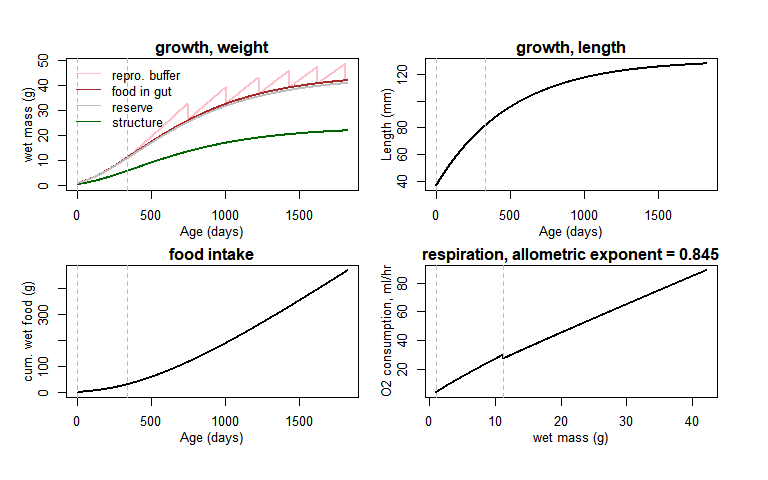# Integration of DEB with the ectotherm model

Finally, we can put the microclimate, heat budget and DEB model together by turning on the DEB model in the NicheMapR ectotherm function and passing it the DEB parameters for the species of interest. Here we simulate Eulamprus quoyii in the location of Townsville, northern Australia, as was done in the Introduction to the NicheMapR ectotherm model vignette.

First, a microclimate must be simulated. The code below specifies the ‘micro_global’ function to run daily for five years in Townsville, with all other settings as default. See the vignette Introduction to the NicheMapR microclimate model for more details on the microclimate model. The ‘micro_global’ function uses a long-term average monthly climatology. The ‘micro_ncep’ function can be used for historical daily weather data globally, and there are other continent-specific functions for simulating microclimate in NicheMapR (‘micro_aust’, ‘micro_usa’, ‘micro_uk’ and ‘micro_nz’).

longlat <- c(146.77, -19.29) # Townsville, northern Australia
nyear <- 5
micro <- micro_global(loc = longlat, timeinterval = 365, nyear = nyear)

Next, load the allstat dataset, choose the species of interest and extract the parameters.

load('allstat.Rda') # load the allstat file

species <- "Eulamprus.quoyii"

allDEB.species<-unlist(labels(allStat$allStat)) # get all the species names allDEB.species<-allDEB.species[1:(length(allDEB.species)-2)] # last two elements are not species species.slot <- which(allDEB.species == species) par.names <- unlist(labels(allStat$allStat[[species.slot]]))
# clear possible missing parameters
if(exists("E.Hj")==TRUE){rm(E.Hj)}
if(exists("E.He")==TRUE){rm(E.He)}
if(exists("L.j")==TRUE){rm(L.j)}
if(exists("T.L")==TRUE){rm(T.L)}
if(exists("T.H")==TRUE){rm(T.H)}
if(exists("T.AL")==TRUE){rm(T.AL)}
if(exists("T.AH")==TRUE){rm(T.AH)}

for(i in 1:length(par.names)){
assign(par.names[i], unlist(allStat$allStat[[species.slot]][i])) } # assign possible missing parameters if(exists("E.Hj")==FALSE){E.Hj <- E.Hb} if(exists("E.He")==FALSE){E.He <- E.Hb} if(exists("L.j")==FALSE){L.j <- L.b} F.m <- p.Am / kap.X # redefining F.m to max possible value z.mult <- 1 # DEB body size scaling parameter # assign missing 5-par thermal response curve parameters if necessary if(exists("T.L")==FALSE){T.L <- CT_min + 273.15} if(exists("T.H")==FALSE){T.H <- CT_max + 273.15} if(exists("T.AL")==FALSE){T.AL <- 5E04} if(exists("T.AH")==FALSE){T.AH <- 9E04} # overwrite nitrogenous waste indices with those of uric acid (currently ammonia by default) n.NC <- 1 n.NH <- 4/5 n.NO <- 3/5 n.NN <- 4/5 Next, as described previously in the Introduction to the NicheMapR ectotherm model vignette, we need to define the morphological, behavioural and physiological parameters for the biophysical models of heat and water exchange. # morph, behav and water loss pct_wet <- 0.2 # % of surface area acting as a free-water exchanger alpha_max <- 0.85 # maximum solar absorptivity alpha_min <- 0.85 # minimum solar absorptivity shape <- 3 # animal shape - 3 = lizard T_RB_min <- 17.5 # min Tb at which they will attempt to leave retreat T_B_min <- 17.5 # min Tb at which leaves retreat to bask T_F_min <- 24 # minimum Tb at which activity occurs T_F_max <- 34 # maximum Tb at which activity occurs T_pref <- 30 # preferred Tb (will try and regulate to this) CT_max <- 40 # critical thermal minimum (affects choice of retreat) CT_min <- 6 # critical thermal maximum (affects choice of retreat) mindepth <- 2 # min depth (node, 1-10) allowed maxdepth <- 10 # max depth (node, 1-10) allowed shade_seek <- 1 # shade seeking? burrow <- 1 # can it burrow? climb <- 0 # can it climb to thermoregulate? minshade <- 0 # min available shade? nocturn <- 0 # nocturnal activity crepus <- 0 # crepuscular activity diurn <- 1 # diurnal activity minshades <- rep(0, nyear * 365) # min available shade? maxshades <- micro$MAXSHADES # max available shade?

Because we are running the DEB model, we need to set the initial state in terms of structure, reserve, maturity and life cycle stage. Here we set it to an embryo but the code for a hatchling or a mature individual is also included.

# DEB initial state

# egg
V_init <- 3e-9
E_init <- E.0 / V_init
E_H_init <- 0
stage <- 0

# hatchling
# V_init <- L.b^3
# E_init <- E.m
# E_H_init <- E.Hb
# stage <- 1

# mature
# V_init <- L.p^3
# E_init <- E.m
# E_H_init <- E.Hp+2
# stage <- 2

Finally, we need to define some reproduction parameters that determine the reproductive mode, clutch size and the breeding season. Here we run the simulation so that the lizard is viviparous. As described in more detail in Schwarzkopf et al. (2016), the viviparous simulation has the eggs developing at the temperature of a thermoregulating adult. Breeding is simulated to occur between the winter and autumnal equinoxes and during this time the ‘batch buffer’ can be filled up to produce eggs. Outside this period, the ‘reproduction buffer’ can be filled but no gonad forms in the animal. See Pecquerie et al. 2009 for more details.

# reproduction parameters
viviparous <- 1 # live bearing (1) or egg laying (0)
clutchsize <- 5 # how many eggs per clutch?
photostart <- 3 # winter solstice is the start of the reproduction cycle
photofinish <- 2 # autumnal equinox is the end of the reproduction cycle

Now the ectotherm function can be called, with all these parameters, including the DEB parameters extracted from the allstat file, passed in.

# run the ectotherm model
ecto<-ectotherm(DEB=1,
viviparous=viviparous,
clutchsize=clutchsize,
z.mult=z.mult,
shape=shape,
alpha_max=alpha_max,
alpha_min=alpha_min,
T_F_min=T_F_min,
T_F_max=T_F_max,
T_B_min=T_B_min,
T_RB_min=T_RB_min,
T_pref=T_pref,
CT_max=CT_max,
CT_min=CT_min,
diurn=diurn,
nocturn=nocturn,
crepus=crepus,
shade_seek=shade_seek,
burrow=burrow,
climb=climb,
mindepth=mindepth,
maxdepth=maxdepth,
minshade=minshade,
pct_wet=pct_wet,
z=z*z.mult,
del_M=del.M,
F_m=F.m,
kap_X=kap.X,
v=v/24,
kap=kap,
p_M=p.M/24,
E_G=E.G,
kap_R=kap.R,
k_J=k.J/24,
E_Hb=E.Hb*z.mult^3,
E_Hj=E.Hj*z.mult^3,
E_Hp=E.Hp*z.mult^3,
E_He=E.He*z.mult^3,
h_a=h.a/(24^2),
s_G=s.G,
T_REF=T.ref,
T_A=T.A,
T_AL=T.AL,
T_AH=T.AH,
T_L=T.L,
T_H=T.H,
E_0=E.0*z.mult^4,
f=f,
d_V=d.V,
d_E=d.E,
d_Egg=d.E,
mu_X=mu.X,
mu_E=mu.E,
mu_V=mu.V,
mu_P=mu.P,
kap_X_P=kap.P,
n_X=c(n.CX,n.HX,n.OX,n.ON),
n_E=c(n.CE,n.HE,n.OE,n.OE),
n_V=c(n.CV,n.HV,n.OV,n.OV),
n_P=c(n.CP,n.HP,n.OP,n.OP),
n_M_nitro=c(n.NC,n.NH,n.NO,n.NN),
L_b=L.b,
V_init=V_init,
E_init=E_init,
E_H_init=E_H_init,
stage=stage,
photostart = photostart,
photofinish = photofinish)

# retrieve output
environ<-as.data.frame(ecto$environ) # behaviour, Tb and environment enbal<-as.data.frame(ecto$enbal) # heat balance outputs
masbal<-as.data.frame(ecto$masbal) # mass balance outputs debout<-as.data.frame(ecto$debout) # DEB model outputs
yearout <- as.data.frame(ecto$yearout) # whole life cycle summary yearsout <- as.data.frame(ecto$yearsout) # annual summaries

The output tables environ, enbal and masbal were retrieved from the model run as done in the Introduction to the NicheMapR ectotherm model vignette. Now the masbal table includes values for all the DEB-based mass flow calculations:

DOY YEAR DAY TIME O2_ml CO2_ml NWASTE_g H2OFree_g H2OMet_g
43789 365 5 1825 12 2.168638 0.9460227 0.0045605 0.0303789 0.0018270
43790 365 5 1825 13 2.068990 0.9025535 0.0043370 0.0288895 0.0017374
43791 365 5 1825 14 2.161036 0.9427071 0.0045433 0.0302642 0.0018201
43792 365 5 1825 15 2.101908 0.9169145 0.0044104 0.0293789 0.0017669
43793 365 5 1825 16 2.184666 0.9530162 0.0045969 0.0306207 0.0018415
43794 365 5 1825 17 2.151508 0.9385521 0.0045218 0.0301208 0.0018115
43795 365 5 1825 18 2.121924 0.9256472 0.0044553 0.0296774 0.0017848
43796 365 5 1825 19 2.099871 0.9160277 0.0044059 0.0293483 0.0017650
43797 365 5 1825 20 2.235681 0.9752727 0.0047135 0.0313978 0.0018883
43798 365 5 1825 21 2.333917 1.0181266 0.0049474 0.0329557 0.0019820
43799 365 5 1825 22 2.361757 1.0302718 0.0050261 0.0334796 0.0020135
43800 365 5 1825 23 2.358250 1.0287427 0.0050324 0.0335217 0.0020160
DryFood_g WetFood_g DryFaeces_g WetFaeces_G Urine_g H2OResp_g
43789 0.0066685 0.0370474 0.0006956 0.0025761 0.0045605 0
43790 0.0063416 0.0352311 0.0006615 0.0024498 0.0043370 0
43791 0.0066434 0.0369076 0.0006929 0.0025664 0.0045433 0
43792 0.0064490 0.0358279 0.0006727 0.0024913 0.0044104 0
43793 0.0067216 0.0373424 0.0007011 0.0025967 0.0045969 0
43794 0.0066119 0.0367327 0.0006896 0.0025543 0.0045218 0
43795 0.0065146 0.0361920 0.0006795 0.0025167 0.0044553 0
43796 0.0064423 0.0357906 0.0006720 0.0024888 0.0044059 0
43797 0.0068922 0.0382900 0.0007189 0.0026626 0.0047135 0
43798 0.0072342 0.0401899 0.0007546 0.0027947 0.0049474 0
43799 0.0073492 0.0408288 0.0007666 0.0028391 0.0050261 0
43800 0.0073584 0.0408802 0.0007675 0.0028427 0.0050324 0
H2OCut_g H2OEye_g H2OBal_g H2OCumBal_g
43789 0.0003365 0 0.0299888 0
43790 0.0003437 0 0.0284948 0
43791 0.0003370 0 0.0298738 0
43792 0.0003414 0 0.0289856 0
43793 0.0003349 0 0.0302318 0
43794 0.0003377 0 0.0297300 0
43795 0.0003399 0 0.0292852 0
43796 0.0003414 0 0.0289552 0
43797 0.0003295 0 0.0310129 0
43798 0.0003119 0 0.0325857 0
43799 0.0002968 0 0.0331238 0
43800 0.0002851 0 0.0331776 0
• DOY - Day of year
• YEAR - Year of simulation
• DAY - Day of simulation
• TIME - Time of day (hours)
• O2_ml - Oxygen consumption rate (ml/h)
• CO2_ml - Carbon dioxide production rate (ml/h)
• NWASTE_g - Nitrogenous waste production (g/h)
• H2OFree_g - Free water (i.e. in food) consumption (g/h)
• H2OMet_g - Metabolic water production (g/h)
• DryFood_g - Dry food consumption (g/h)
• WetFood_g - Wet food consumption (g/h)
• DryFaeces_g - Dry faeces production (g/h)
• WetFaeces_g - Wet faeces production (g/h)
• Urine_g - Urine production (NWASTE plus water) (g/h)
• H2OResp_g - Respiratory water loss (g/h)
• H2OCut_g - Cutaneous water loss (g/h)
• H2OEye_g - Ocular water loss (g/h)
• H2OBal_g - Instantaneous water balance (g/h)
• H2OCumBal_g - Cumulative water balance (g)

There is also now the output table debout which contains the following variables:

DOY YEAR DAY TIME STAGE V E E_H L_W WETMASS
43789 365 5 1825 12 3 22.16155 5952.236 14380.05 127.9265 56.31503
43790 365 5 1825 13 3 22.16165 5952.236 14380.05 127.9267 56.28339
43791 365 5 1825 14 3 22.16176 5952.236 14380.05 127.9269 56.25024
43792 365 5 1825 15 3 22.16187 5952.236 14380.05 127.9271 56.21805
43793 365 5 1825 16 3 22.16198 5952.236 14380.05 127.9273 56.18450
43794 365 5 1825 17 3 22.16209 5952.236 14380.05 127.9275 56.15149
43795 365 5 1825 18 3 22.16220 5952.236 14380.05 127.9277 56.11897
43796 365 5 1825 19 3 22.16230 5952.236 14380.05 127.9279 56.08680
43797 365 5 1825 20 3 22.16241 5952.236 14380.05 127.9281 56.05238
43798 365 5 1825 21 3 22.16253 5952.236 14380.05 127.9284 56.01625
43799 365 5 1825 22 3 22.16265 5952.236 14380.05 127.9286 55.97954
43800 365 5 1825 23 3 22.16277 5952.236 14380.05 127.9288 55.94278
WETGONAD WETGUT PCT_DESIC E_R E_B BREEDING
43789 9.183298 1.945757 0 8593.716 46661.21 1
43790 9.183514 1.913686 0 8604.872 46661.21 1
43791 9.183736 1.880089 0 8616.560 46661.21 1
43792 9.183948 1.847475 0 8627.906 46661.21 1
43793 9.184165 1.813483 0 8639.732 46661.21 1
43794 9.184374 1.780045 0 8651.364 46661.21 1
43795 9.184576 1.747100 0 8662.826 46661.21 1
43796 9.184772 1.714520 0 8674.160 46661.21 1
43797 9.184977 1.679665 0 8686.286 46661.21 1
43798 9.185188 1.643080 0 8699.013 46661.21 1
43799 9.185398 1.605914 0 8711.943 46661.21 1
43800 9.185602 1.568701 0 8724.889 46661.21 1
PREGNANT V_BABY E_BABY H_S P_SURV
43789 1 0.1231422 65763.67 1.09e-05 0.8680813
43790 1 0.1235692 65500.57 1.09e-05 0.8680718
43791 1 0.1240173 65226.40 1.09e-05 0.8680624
43792 1 0.1244531 64961.66 1.09e-05 0.8680529
43793 1 0.1249081 64687.20 1.09e-05 0.8680434
43794 1 0.1253565 64418.67 1.09e-05 0.8680339
43795 1 0.1257991 64155.51 1.09e-05 0.8680244
43796 1 0.1262375 63896.62 1.09e-05 0.8680149
43797 1 0.1267074 63621.13 1.09e-05 0.8680055
43798 1 0.1272015 63333.62 1.09e-05 0.8679960
43799 1 0.1277045 63043.25 1.09e-05 0.8679865
43800 1 0.1282091 62754.22 1.09e-05 0.8679770
• DOY - Day of year
• YEAR - Year of simulation
• DAY - Day of simulation
• TIME - Time of day (hours)
• Stage - Life cycle stage (0=embryo, 1=juvenile, etc.)
• V - Structural volume (cm3)
• E - Reserve density (J/cm3)
• E_H - Maturity state (J)
• LENGTH - Physical length (mm)
• WETMASS - Wet mass total (g)
• WETGONAD - Wet mass of gonad (batch and reproduction buffers) (g)
• WETGUT - Wet mass of food in gut (g)
• PCT_DESIC - % desiccated
• CUMREPRO - Energy in reproduction buffer (J)
• CUMBATCH - Energy in batch for egg production (J)
• BREEDING - Breeding state (1=breeding, 0=not breeding)
• PREGNANT - Pregnant? (only if viviparous) (0 or 1)
• V_BABY - Structure of baby (cm3) (only if viviparous and pregnant)
• E_BABY - Reserve density of baby (J/cm3) (only if viviparous and pregnant)
• H_S - Hazard rate (1/h)
• P_SURV - Survival probability (ageing and mortality rates combined) (-)

Figure 6 shows plot of the wet mass, using the same colour scheme to represent the different biomass components as in Figure 2.

par(mfrow = c(1,1))
plot(seq(1, ndays * 24) / 24, debout$WETMASS, type = 'l', xlab = 'date', ylab = paste0('wet mass (g)'), col = 'pink', lwd = 2, ylim = c(0, max(debout$WETMASS)))
points(seq(1, ndays * 24) / 24, debout$V, type = 'l', xlab = 'date', ylab = paste0('wet mass (g)'), col = 'dark green', lwd = 2) points(seq(1, ndays * 24) / 24, debout$WETMASS-debout$WETGONAD, type = 'l', lwd = 2, col = 'brown') points(seq(1, ndays * 24) / 24, debout$WETMASS-debout$WETGONAD-debout$WETGUT,
type = 'l', lwd = 2, col = 'grey')
abline(v = (seq(1, ndays * 24) / 24)[which(debout$E_H>E.Hb)], lty = 2, col = 'grey') abline(v = (seq(1, ndays * 24) / 24)[which(debout$E_H>E.Hp)], lty = 2, col = 'grey')
legend(1200, max(debout$WETMASS) * 0.3, c('repro. buffer', 'food in gut', 'reserve', 'structure'), lty = rep(1, 4), col = c("pink", "brown", "grey", "dark green"), bty = 'n') text(0, max(debout$WETMASS) * 1, labels = "embryo", cex = 0.85)
text((which(debout$E_H > E.Hp) - which(debout$E_H > E.Hp) * .5) / 24 ,
max(debout$WETMASS) * 1, labels = "immature", cex = 0.85) text(which(debout$E_H > E.Hp) * 1.2 / 24, max(debout\$WETMASS) * 1,
labels = "adult", cex = 0.85)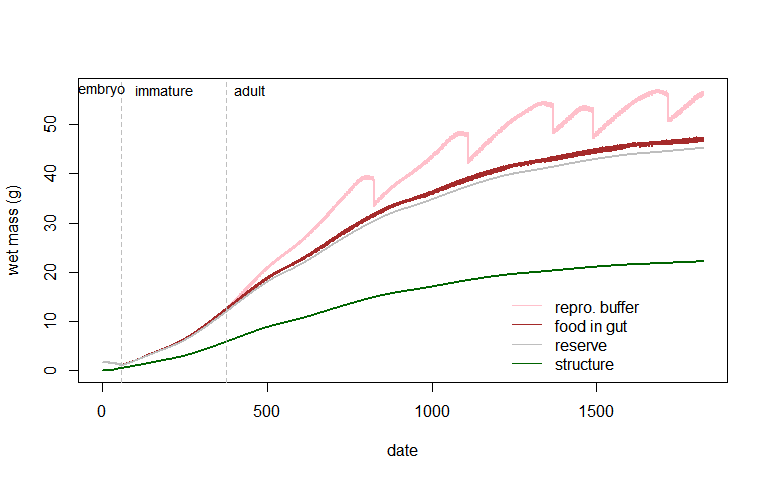DEB model prediction of wet weight through time of the lizard Eulamprus quoyii growing under constant ad libitum under field conditions in Townsville, Queensland Australia, partitioning total biomass mass into structure, reserve, reproduction buffer and gut contents.

And finally there are tables summarising various life history events per year and over the entire simulation – the yearsout and yearout tables.

Here is the yearsout table, and a description of the different outputs.

YEAR MaxStg MaxWgt MaxLen Tmax Tmin MinRes
1 1 12.03274 81.00165 37.20724 15.38216 1
2 3 35.24771 107.58867 37.15654 15.39689 1
3 3 48.56033 119.77345 37.17475 15.40700 1
4 3 54.61817 125.36272 37.19098 15.40805 1
5 3 57.00918 127.92882 37.19691 15.41132 1
MaxDes MinShade MaxShade MinDep MaxDep Bsk Forage
0 0 90 0 -20 684 549
0 0 90 0 -20 1427 1026
0 0 90 0 -20 2201 1447
0 0 90 0 -20 3001 1856
0 0 90 0 -20 3819 2261
Dist Food Drink NWaste Faeces O2 Clutch Fec
0 10.62230 0 5.902718 1.107955 2357.776 0 0
0 41.23043 0 24.210403 4.300525 10135.870 0 0
0 84.45692 0 52.117719 8.809248 22673.186 1 5
0 134.21745 0 85.429900 13.999502 38002.576 3 15
0 187.10535 0 121.428277 19.515955 54743.350 5 25
CauseDeath tLay tEgg tStg1 tStg2 tStg3 mStg1 mStg2 mStg3 surviv deathstage
0 0 1 59 0 0 1.092505 0 0.00000 0.9989563 -1
0 0 1 59 0 10 1.092505 0 12.42509 0.9923237 -1
0 94 1 59 0 173 1.092505 0 38.27809 0.9737859 -1
0 275 1 59 0 173 1.092505 0 38.27809 0.9350395 -1
0 259 1 59 0 173 1.092505 0 38.27809 0.8679770 -1
• YEAR - Year of simulation
• MaxStg - Maximum stage reached in the year
• MaxWgt - Maximum weight reached in the year (g)
• MaxLen - Maximum length in the year (mm)
• Tmax - Maximum annual body temperature (°C)
• Tmin - Minimum annual body temperature (°C)
• MinRes - Minimum annual reserve density (J/cm3)
• MaxDes - Maximum annual desiccation level (% of normal wet body mass)
• MinShade - Minimum annual shade selected
• MaxShade - Maximum annual shade selected
• MinDep - Minimum annual depth selected (cm)
• MaxDep - Maximum annual depth selected (cm)
• Bsk - Annual basking hours
• Forage - Annual foraging hours
• Dist - Annual distance travelled (flying insect) (km)
• Food - Annual food eaten (g)
• Drink - Annual water drunk (g)
• NWaste - Annual nitrogenous waste (g)
• Faeces - Annual faeces production (g)
• O2 - Annual O2 production (ml)
• Clutch - Annual clutches (#)
• Fec - Annual fecundity (#)
• CauseDeath - Cause of death, 0=no death, 1=cold, 2=heat, 3=desicc., 4=starv., 5=ageing
• tLay - Day of year at which eggs laid
• tEgg - Day of year entering egg stage
• tStg1-tStg8 - Day of year entering life cycle stages 1-8
• mStg1-mStg8 - Mass entering life cycle stages 1-8 (g)
• surviv - Survival probability at end of given year
• deathstage - Life stage at which death occurred

And here is the yearout table.

DEVTIME BIRTHDAY BIRTHMASS MONMATURE MONREPRO LENREPRO FECUNDITY
58 10 0 12.29508 56.36066 127.3189 25
CLUTCHES ANNUALACT MINRESERVE LASTFOOD TOTFOOD MINTB MAXTB
5 6080 5952.236 919.4089 1020013 15.38216 37.20724
Pct_Des LifeSpan GenTime R0 rmax Length
0 0 3.473009 0.4952959 -0.2023029 55.94278
• DEVTIME - Development time (days)
• BIRTHDAY - Birth day (day of year)
• BIRTHMASS - Mass at birth (g)
• MONMATURE - Months to maturity
• LENREPRO - Length (mm) at first reproduction
• FECUNDITY - Total fecundity
• CLUTCHES - Total clutches
• MINRESERVE - Minimum reserve density (J/cm3)
• LASTFOOD - Food in last year (kg)
• TOTFOOD - Total food eaten (kg)
• MINTB - Minimum body temperature (°C)
• MAXTB - Maximum body temperature (°C)
• Pct_Des - Maximum level of desiccation (/%)
• LifeSpan - Maximum life span (days)
• GenTime - Generation time (years)
• R0 - Net reproductive rate
• rmax - Intrinsic rate of increase
• LENGTH - Maximum length (mm)

You can see that the yearout table contains predictions of the net reproductive rate and intrinsic rate of increase, which are computed based on a life table of survival probabilities and fecundities per year and can be mapped as an ultimate summary of the suitability of a given location (see e.g. Kearney 2012).

The survival probabilities are based on the combination of the DEB-based aging model as well as the hourly mortality probabilities for the animal when it is active (parameter m_a, by default set to 1e-4), when it is inactive (m_i, by default set to zero) and in its first year as a hatchling (m_h, 0.5 by default). These ‘vital rate’ statistics can be used as the basis of further population modelling and to indirectly infer maximum tolerable mortality rates from processes other than senescence.